# Power-law for axon diameters at branch point

## Abstract

### Background

Axon calibers vary widely among different animals, neuron classes, and even within the same neuron. What determines the diameter of axon branches?

### Results

We pursue the hypothesis that the axon caliber has evolved to minimize signal propagation delays, while keeping arbor volume to a minimum. For a general cost function, we show that the optimal diameters of mother and daughter branches at a bifurcation satisfy a power law. The derivation relies on the fact that the axon conduction speed scales as a power of axon diameter. Although available data are consistent with the law, there is a large spread in the data. Future experimental tests will determine whether this spread is due to biological variability or measurement error.

### Conclusions

Minimization of arbor volume and signal propagation delay may have been an important factor in the evolution of the brain.

## Background

Multi-cellular organisms have solved the problem of efficient transport of nutrients and communication between their body parts by evolving spectacular networks: trees, blood vessels, bronchs, and neuronal arbors. These networks consist of segments bifurcating into thinner and thinner branches. Understanding of branching in transport networks has been advanced through the application of the optimization theory [1, 2] and references therein). Here we apply the optimization theory to explain the caliber of branching segments in communication networks, i.e. neuronal axons.

Axons in different organisms vary in caliber from 0.1 μm (terminal segments in neocortex) to 1000 μm (squid giant axon) . What factors could be responsible for such variation in axon caliber? According to the experimental data  and cable theory , thicker axons conduct action potential faster, leading to shorter reaction times and, perhaps, quicker thinking. This increases evolutionary fitness or, equivalently, reduces costs associated with conduction delays. So, why not make all the axons infinitely thick? It is likely that thick axons are evolutionary costly because they require large amount of cytoplasm and occupy valuable space . Then, is there an optimal axon caliber, which minimizes the combined cost of conduction delays and volume?

In this paper we derive an expression for the optimal axon diameter, which minimizes the combined cost of conduction delay and volume. Although the relative cost of delay and volume is unknown, we use this expression to derive a law describing segment calibers of branching axons with no free parameters. We test this law on the published anatomical data and find a satisfactory agreement.

## Results and Discussion

Although our theory holds for a rather general class of cost functions (see Methods), we start, for the sake of simplicity, by deriving the branching law in a special case of a linear cost function. Detrimental contribution to fitness,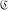, of an axon segment of length, L, can be represented as the sum of two terms, one proportional to the conduction delay along the segment, T, and the other to the segment volume, V: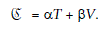(1)

Here, α and β are unknown but constant (among axons of a given class) coefficients which reflect the relative contribution to the fitness cost of the signal propagation delay and the axon volume.

We look for the axon caliber d that minimizes the cost function. To do this, we rewriteas a function of d by noticing the following relations: i) Volume,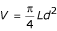; ii) Time delay,; iii) Conduction velocity is s = kd for myelinated axons , where k is a constant coefficient (for non-myelinated axons, see Methods):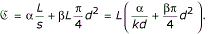(2)

This cost function contains two terms, which have opposite dependence on d, and has a minimum, Fig. 1.

Next, by setting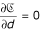we find that the cost is minimized by the following axon caliber: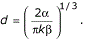(3)

The utility of this result may seem rather limited because the relative cost of time delays vs. volume, α /β, is unknown.

However, we can apply this result to axon branching and arrive at a testable prediction about the relationship among branch diameters without knowing the relative cost. To do this we write the cost function for a bifurcation consisting of three segments, Fig. 2: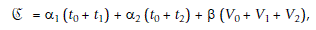(4)

where t0, t1, and t2 are the conduction delays along segments 0, 1, and 2 respectively. Coefficients α1 and α2 represent relative costs of conduction delays for synapses located on the two daughter branches and may be different. We group the terms corresponding to the same segment together: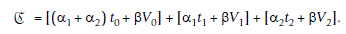(5)

We look for segment diameters, which minimize this cost function. To do this we make the dependence on the diameters explicit and differentiate in respect to them. Because each term in Eq. (5) depends on the diameter of only one segment the variables separate and we arrive at expressions analogous to Eq. (3):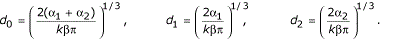(6)

It is easy to see that these diameters satisfy the following branching law: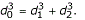(7)

Similar expression can be derived for non-myelinated axons (see Methods). In this case, the conduction velocity scales with the square root of segment diameter , resulting in a branching exponent of 2.5.

Although the branching law is independent of the relative costs of volume and propagation delay, axon diameters depend on the relative values of α and β. Axons with higher premium on propagation delays (greater α) are expected to be thicker, while axons with higher premium on volume (greater β) are expected to be thinner. Yet the law still holds. For example, if propagation delays to synapses on daughter branch 1 are costlier than to synapses on branch 2, branch 1 must be thicker than branch 2. The derivation of the branching law relies only on the assumptions about the scaling of the two costs with diameter. Although existing evidence seems to support both assumptions, more experimental testing is desirable. Also, the range of validity of Eq. (7) is restricted by the biophysical limits on the minimal axon caliber .

We note that expressions analogous to Eq. (7) have been derived for blood vessels, tree branches and bronchs by balancing metabolic cost of pumping viscous fluid and volume cost [14, 15]. It is hard to see why the viscous fluid argument is applicable to axons and dendrites  if their ends are sealed. Rall  has derived a similar law for branching dendrites by postulating impedance matching: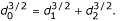(8)

However, the main purpose of Rall's law was to simplify calculations of dendritic conduction rather than to explain the actual branch caliber measurements. Axon diameter may also be dictated by the need to transport material to the synaptic terminals [17, 18]. In this case, one would expect a power law with exponent of 2 .

We test our branching law, Eq. (7), by comparing it with data obtained from myelinated motor fibers of the cat , Fig. 3. Data points represent 63 branch points for which all three axon calibers were measured with light microscopy. Eq. (7) predicts that data points should fall on the line described by: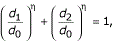(9)

where exponent η = 3. Despite the large spread in the data it is consistent with our predictions. In fact, the best fit exponent, η = 2.57, is closer to our prediction than to Rall's law, η = 1.5. We also show the histogram of the exponents η obtained for each of 63 branch points from the same data set, Fig. 4. The average exponent, η = 2.67, is much closer to our predicted value for myelinated axons, η = 3, than to Rall's law, η = 1.5.

Analysis of the experimental data reveals a large spread in the values of the exponent, η. This spread may arise from the biological variability in the axon diameters, other factors influencing axon diameters, or measurement errors due to the finite resolution of light microscopy. Although, at this point, we cannot distinguish between these causes, we performed a simulation showing that a reasonable measurement error is sufficient to account for the spread.

First, based on the experimental data , we generate a set of diameters d0, d1 and d2 at branch points, which satisfy Eq. (7). We do this by taking all diameter pairs at branch point from the experimental data and calculating the value of the third diameter according to Eq. (7). Next we simulate the experimental data by adding Gaussian noise to all branch diameters, and calculate the probability distribution for the exponent η resulting from this procedure. The line in Fig. 4 shows that the spread in the histogram of branching exponent could be explained by Gaussian measurement error with standard deviation of 0.4 μm (p > 0.23, Kolmogorov-Smirnov test). This value of standard deviation is consistent with 0.5 μm precision with which diameter measurements are reported in .

We note that a similar branching law could apply to dendrites. There, similar to non-myelinated axons, time delay or attenuation of passively propagating signals scales as one over the square root of diameter. This leads to a branching law with exponent of 5/2, which agrees with the measurements done on pyramidal, Purkinje, granule, and stellate cells but not on motorneurons . However, the presence of reflections from branch points and active conductances is likely to complicate the picture.

Previously, Cherniak et al.  have fitted diameters from a pool of myelinated and non-myelinated axons, and dendrites with Eq. (9). They also found the best-fit exponent η = 3 and a large spread in the data. Unfortunately, they did not attempt to fit the data separately for myelinated and non-myelinated axons.

We hope that future experiments will thoroughly test the branching law. One crucial experiment could combine the measurement of the conduction speed exponent, ν, and the branching exponent, η, in the same axons. Another test would be measuring the branching exponent separately for myelinated and non-myelinated axons. We predict different values for the branching exponent because of the difference in ν between myelinated and non-myelinated axons. Also, improving the relative measurement error should determine whether the observed spread in the existing data is biological reality or a measurement artifact. This can be done either by increasing the measurement precision, e.g. by using transmission or scanning electron microscopy, or by measuring thicker axons, e.g. by using marine animals.

## Conclusions

Starting with the hypotheses that axon arbors had been optimized in the course of evolution for fast signal conduction while keeping arbor volume to a minimum we derived a branching law that relates segment diameters at a branch point. The derivation was done for the cost function of a general form, and relies only on the scaling of signal propagation velocity with the axon caliber. Existing experimental data on myelinated axons is consistent with the law but shows significant spread in the branching exponent. The observed spread may be due to the measurement error or biological variability.

If future experiments reveal that the spread in the branching exponent is real, meaning that the law poorly describes individual branch points, the law can still be used in average sense. For example, it could be used to estimate costs of many branching axons in the theory of cortical maps  or in the derivation of larger scale allometric relations .

If future experiments confirm the validity of the branching law and eliminate the spread in the data, this law can be used to predict branch diameters. Alternatively, measuring branch diameters would reveal the scaling exponents, which can be used to infer the mechanism of conduction in a particular case. Most importantly, this law provides a deep insight into the design of the nervous system by revealing constraints on wiring, i.e. axons and dendrites responsible for most of the brain volume. The branching law is a quantitative expression of the trade-off between space and time constraints. Finally, optimization approach to brain design helps formulating structure-function relationships, which should provide insight into brain function.

## Methods

The detrimental contribution of an axon arbor to the evolutionary fitness can be quantified by the cost,. We postulate that the cost function,, is a monotonically increasing function of the total axon volume per neuron, V, and all signal propagation delays, T j , from soma to j-th synapse, where j = 1,2,3,...: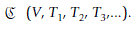(10)

Below we show that this rather general cost function (along with biophysical properties of axons) is minimized when axon caliber satisfies the following branching law: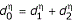(11)

with branching exponent η = 3 for myelinated and η = 2.5 for non-myelinated axons.

Although we derive Eq. (11) for a single branch point, our theory can be trivially extended to more complex arbor topologies. We rewrite the cost function,, in terms of volume contributions, V i , of i-th axon segment to the total volume of the axon arbor, V, and signal propagation delay, t i , occurred along i-th axon segment. The cost function reduces to: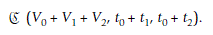(12)

Next, we express volume and signal propagation delay of each segment as a function of segment diameter. The volume of each cylindrical segment is given by: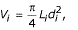(13)

where L i and d i are segment length and diameter, correspondingly. Signal propagation delay, t i , is given by the ratio of segment length, L i , and signal speed, s i . Signal speed along axon segment, in turn, depends on its diameter as: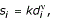(14)

where ν = 1 for myelinated and ν = 0.5 for non-myelinated fibers . As a result propagation delay along segment i is: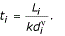(15)

Substituting Eqs. (13), (15) into the cost function, Eq. (12), we find the dependence of the cost function on segment diameters,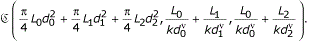(16)

To find the diameters of all segments, which minimize the cost function, we calculate its partial derivatives with respect to all segment diameters and set them to zero: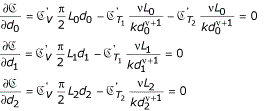(17)

By solving these equations we find the optimal segment diameters: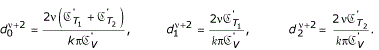(18)

These equations imply that the cost function is minimized when the segment diameters at a branch point satisfy the following expression (independent of the particular form of the cost function, which enters Eq. (18) through the partial derivatives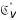,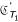, and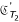):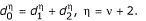(19)

## References

1. 1.

Brown James H., West Geoffrey B., Santa Fe Institute (Santa Fe N.M.): Scaling in biology. 2000, Oxford ; New York, Oxford University Press, xiii, 352.

2. 2.

Weibel Ewald R.: Symmorphosis: on form and function in shaping life. 2000, Cambridge, Mass. ; London, Harvard University Press, xiii, 263.

3. 3.

Purves Dale: Neuroscience. 1997, Sunderland, Mass., Sinauer Associates, 1 v. (various pagings).

4. 4.

Hursh JB: Conduction velocity and diameter of nerve fibers. Amer J Physiol. 1939, 127: 131-139.

5. 5.

Rushton WAH: A theory of the effects of fibre size in medullated nerve. J Physiol. 1951, 115: 101-122.

6. 6.

Bullock Theodore Holmes, Horridge G. Adrian: Structure and function in the nervous systems of invertebrates. 1965, San Francisco,, W. H. Freeman, 2 v. (xx, 1719 ).

7. 7.

Hodgkin AL: A note on conduction velocity. J Physiol. 1954, 125: 221-224.

8. 8.

Cajal S.R.y: Texture of the Nervous System of Man and the Vertebrates. 1999, New-York, Springer, 1: 631.

9. 9.

Chklovskii DB, Schikorski T, Stevens CF: Wiring optimization in cortical circuits. Neuron. 2002, 34: 341-347.

10. 10.

Cherniak C, Changizi M, Kang DW: Large-scale optimization of neuron arbors. Phys Rev E. 1999, 59: 6001-6009. 10.1103/PhysRevE.59.6001.

11. 11.

Mitchison G: Neuronal branching patterns and the economy of cortical wiring. Proc R Soc Lond B Biol Sci. 1991, 245: 151-158.

12. 12.

Pumphrey RJ, Young JZ: The rates of conduction of nerve fibres of various diameters in cephalopods. J Exper Biol. 1938, 15: 453-466.

13. 13.

Faisal A, White J, Laughlin S: Personal communication. 2003

14. 14.

Murray CD: The physiological principle of minimum work. I. The vascular system and the cost of blood volume. PNAS. 1926, 12: 207-214.

15. 15.

Murray CD: A relationship between circumference and weight in trees and its bearing on branching angles. J Gen Physiol. 1927, 10: 725-729.

16. 16.

Rall W: Branching dendritic trees and motoneuron membrane resistivity. Exp Neurol. 1959, 1: 491-527.

17. 17.

Hsu A, Tsukamoto Y, Smith RG, Sterling P: Functional architecture of primate cone and rod axons. Vision Res. 1998, 38: 2539-2549. 10.1016/S0042-6989(97)00370-2.

18. 18.

Sterling P: Personal communication.

19. 19.

Hillman DE: Neuronal shape parameters and substructures as a basis of neuronal form. The neurosciences: Fourth Study Program. Edited by: Schmitt FO and Worden FG. 1979, Cambridge, MIT Press, 477-498.

20. 20.

Adal MN, Barker D: Intramuscular branching of fusimotor fibers. J Physiol. 1965, 177: 288-299.

21. 21.

Changizi Mark A.: The brain from 25,000 feet: high level explorations of brain complexity, perception, induction, and vagueness. Synthese library ; v. 317. 2003, Dordrecht ; Boston, Kluwer Academic Publishers

## Acknowledgments

D.C. is grateful to Leo and Boris Shklovskii for helpful discussions. This work was supported by the David and Lucile Packard Foundation and the Lita Annenberg Hazen Foundation.

## Author information

Authors

### Corresponding author

Correspondence to Dmitri B Chklovskii.

## Authors’ original submitted files for images

Below are the links to the authors’ original submitted files for images.

## Rights and permissions

Reprints and Permissions

Chklovskii, D.B., Stepanyants, A. Power-law for axon diameters at branch point. BMC Neurosci 4, 18 (2003). https://0-doi-org.brum.beds.ac.uk/10.1186/1471-2202-4-18